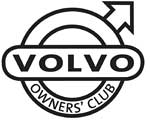# Volvo 940 960 VIN Plates

1800  |  200  |  300  |  400  |  700  |  850 - 900  |  40/50  |  60  |  70  |  80  |  90  |  XC90

This information will help you to understand the information on the VIN plate of your Volvo car.

Click on the model series number above

Example VIN Number:

YV1X44872R1123456

YV1 X44 87 2 R 1 123456
Manufacturer Code Vehicle Type Engine Type Gearbox Model Year Factory Code Chassis number

Manufacturer Code
YV1 = cars YV2 = trucks YV3 = buses YV4 = multipurpose 4V1 = trucks 4V2 = 4V3 =
4V4 = trucks 4V5 = trucks 4V6 = 4VL = 4VM = 4VZ = MHA = PT. Central Sole Agency

Engine Type
20 = B200G 21 = B200FT 22 = B200GT/td> 25 = 204FT 27 = B200F 74 = D24TIC 80 = B230G 81 = B230FK 82 = B230GT W/O EGR, W/O TWC 83 = B230FD Pulsair + EGR 84 = B230GK
85 = B230FB 86 = B230FT EGR, TWC 87 = B230FT W/O EGR, TWC 88 = B230F 92 = B6244S 93 = B6254S EGR, TWC 95 = B6304S TWC 96 = B6304S TWC 98 = B6304GS W/O TWC 99 = B6254S W/O EGR, W/O TWC ---

Gearbox
1 = M90 2 = M46 3 = M47 5 = ZF22 6 = "AW" LOCK-UP 7 = "AW" W/O LOCK-UP

Model Year
R = 1994 S = 1995 T = 1996 V = 1997 W = 1998

Factory Code
0 = Sweden, Kalmar Plant 1 = Sweden, Torslanda Plant VCT 21(Volvo Torslandaverken) (Gothenburg) 2 = Belgium, Ghent Plant VCG 22 3 = Canada, Hatdfax Plant 4 = Italy, - Bertone models 240 5 = Malaysia, 6 = Australia,
7 = Indonesia, A = Sweden, Uddevalla Plant (Volvo Cars/TWR (Tom Walkinshaw Racing)) B = Italy, - Bertone Chongq 31 D = Italy, - Bertone models 780 E = Singapore, F = The Netherlands, Born Plant (NEDCAR) J = Sweden, Uddevalla Plant VCU 38 (Volvo Cars/ Pininfarina Sverige AB)

Model Year From 1980
A = 1980 B = 1981 C = 1982 D = 1983 E = 1984 F = 1985 G = 1986 H = 1987 J = 1988 K = 1989 L = 1990 M = 1991
N = 1992 P = 1993 R = 1994 S = 1995 T = 1996 V = 1997 W = 1998 X = 1999 Y = 2000 1 = 2001 2 = 2002 3 = 2003
4 = 2004 5 = 2005 6 = 2006 7 = 2007 8 = 2008 9 = 2009 A = 2010 (code restarted) B = 2011 C = 2012 D = 2013 E = 2014 ---

### 940

Location of the Chassis/VIN Plate
On a riveted metal plate on the nearside front inner wing. The number shows Volvo security marks.

Visible VIN
Viewed through the near side of the windscreen on a black metal plate.

Stamped Number
On the centre of the of bulkhead.

Location of Engine Number
On the top rear offside of the block on a horizontal lug in a dot matrix format.

### 960

Location of the Chassis/VIN Plate
On the offside of the slam panel.

Stamped Number
Stamped into the offside drivers 'B' Post adjacent to the door catch. Security marks stamped at the beginning and end of the number.

Location of the Engine Number
On the nearside center of the block.